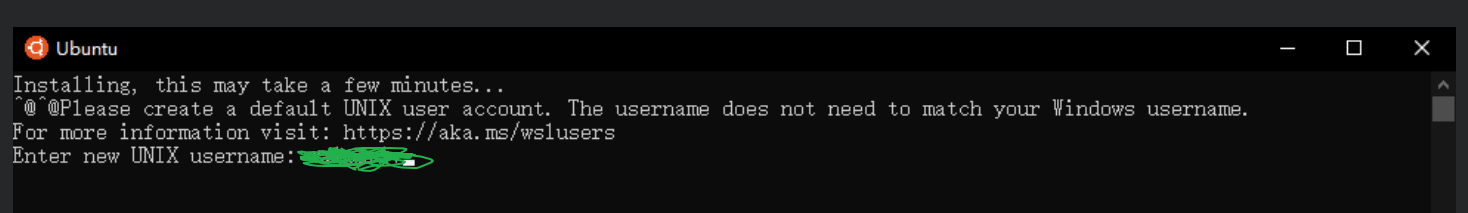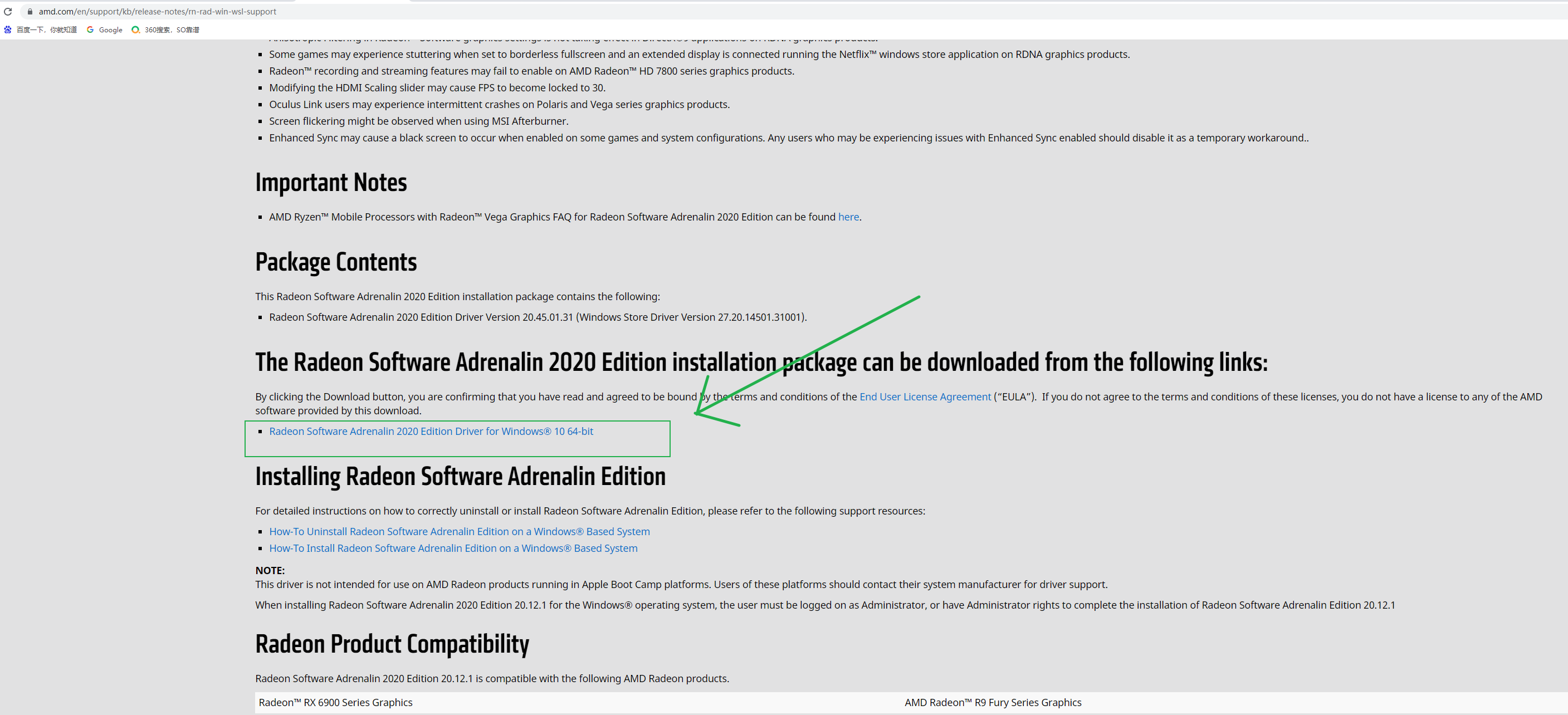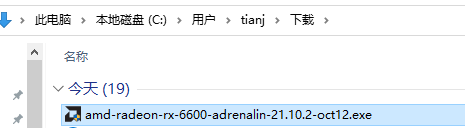# 【AMD显卡在WIndow10/11部署带GPU支持的深度学习环境(Tensorflow-DirectML篇)】CPU：i5 12600KF(目前的神卡，干翻上一代i9 K)

A：搭建WSL2：``````Enable-WindowsOptionalFeature -Online -FeatureName VirtualMachinePlatform
``````

``````Enable-WindowsOptionalFeature -Online -FeatureName Microsoft-Windows-Subsystem-Linux

``````PS：我上面的Terminal是不小心复制进去的，不要在意，回车就完事，反正识别不了。

``````wsl --set-version Ubuntu 2

````````````wsl -l

``````

``````wsl --set-version Ubuntu 2

``````

https://walterlv.blog.csdn.net/article/details/101508601OK，以上就是安装WSL2所有步骤，也适合安装N卡版的，那个安装更简单，弄好直接可以使用原版的TF GPU或者Pytorch GPU而不需要安装DirectML版

Flag1:https://dl.3dmgame.com/patch/89066.html

``````我们的tf dml支持的Python版本：
Python x86-64 3.5, 3.6, or 3.7
``````

https://docs.conda.io/en/latest/miniconda.html

``````pip install tensorflow-directml==1.15.5
``````

Tensorflow 和 Keras 版本对应关系
https://blog.csdn.net/douzi949389/article/details/108146536

``````pip install keras==2.3.1
``````

``````import tensorflow as tf
tf.test.is_gpu_available()
print(tf.test.is_gpu_available(cuda_only=False) )
``````

https://github.com/microsoft/DirectML/tree/master/TensorFlow

``````goto Flag1
``````

PS：
RX6600的小伙伴SDK直接安装这个：https://docs.microsoft.com/zh-cn/windows/ai/directml/gpu-tensorflow-windows

Original: https://blog.csdn.net/weixin_44029053/article/details/121581870
Author: superowner001
Title: 【AMD显卡在WIndow10/11部署带GPU支持的深度学习环境(Tensorflow-DirectML篇)】

## 用法介绍

einops是一个简洁优雅操作张量的库，并且支持对numpy，pytorch，tensorflow中的张量进行操作，该库最大的优点是函数的使用逻辑清晰明了，其中中常用的三个函数分别是rearrange，repeat，reduce。

• rearrange: 用于对张量的维度进行重新变换排序，可用于替换pytorch中的reshape，view，transpose和permute等操作
• repeat: 用于对张量的某一个维度进行复制，可用于替换pytorch中的repeat
• reduce: 类似于tensorflow中的reduce操作，可以用于求平均值，最大最小值的同时压缩张量维度

einops中rearrange，repeat，reduce的函数细节介绍如下所示

def rearrange(inputs, pattern, **axes_lengths)⟶ \longrightarrow ⟶ transform_inputs

• inputs (tensor): 表示输入的张量
• pattern (str): 表示张量维度变换的映射关系
• axes_lengths**: 表示按照指定的规格形式进行变换

def repeat(inputs, pattern, **axes_lengths)⟶ \longrightarrow ⟶ transform_inputs

• inputs (tensor): 表示输入的张量
• pattern (str): 表示张量按照某个维度复制的映射关系
• axes_lengths**: 表示按照指定的规格形式进行复制

def reduce(inputs, pattern, reduction, **axes_lengths)⟶ \longrightarrow ⟶ transform_inputs

• inputs (tensor): 表示输入的张量
• pattern (str): 表示张量执行某种运算做操作后维度变换的映射关系
• reduction (str): 表示运算操作的类型，分别有'max'，'min'，'sum'，'mean'，'prod'
• axes_lengths**: 表示按照指定的规格形式进行运算操作

## 代码示例

einops库中rearrange函数的代码示例如下所示

``````
>>> images = [np.random.randn(30, 40, 3) for _ in range(32)]

>>> rearrange(images, 'b h w c -> b h w c').shape
(32, 30, 40, 3)

>>> rearrange(images, 'b h w c -> (b h) w c').shape
(960, 40, 3)

>>> rearrange(images, 'b h w c -> h (b w) c').shape
(30, 1280, 3)

>>> rearrange(images, 'b h w c -> b c h w').shape
(32, 3, 30, 40)

>>> rearrange(images, 'b h w c -> b (c h w)').shape
(32, 3600)

>>> rearrange(images, 'b (h1 h) (w1 w) c -> (b h1 w1) h w c', h1=2, w1=2).shape
(128, 15, 20, 3)

>>> rearrange(images, 'b (h h1) (w w1) c -> b h w (c h1 w1)', h1=2, w1=2).shape
(32, 15, 20, 12)
``````

einops库中repeat函数的代码示例如下所示

``````
>>> image = np.random.randn(30, 40)

>>> repeat(image, 'h w -> h w c', c=3).shape
(30, 40, 3)

>>> repeat(image, 'h w -> (repeat h) w', repeat=2).shape
(60, 40)

>>> repeat(image, 'h w -> h (repeat w)', repeat=3).shape
(30, 120)

>>> repeat(image, 'h w -> (h h2) (w w2)', h2=2, w2=2).shape
(60, 80)

>>> downsampled = reduce(image, '(h h2) (w w2) -> h w', 'mean', h2=2, w2=2)
>>> repeat(downsampled, 'h w -> (h h2) (w w2)', h2=2, w2=2).shape
(30, 40)
``````

einops库中reduce函数的代码示例如下所示

``````>>> x = np.random.randn(100, 32, 64)

>>> y = reduce(x, 't b c -> b c', 'max')

>>> y = reduce(x, 'time batch channel -> batch channel', 'max')
>>> x = np.random.randn(10, 20, 30, 40)

>>> y1 = reduce(x, 'b c (h1 h2) (w1 w2) -> b c h1 w1', 'max', h2=2, w2=2)

>>> y2 = rearrange(y1, 'b (c h2 w2) h1 w1 -> b c (h1 h2) (w1 w2)', h2=2, w2=2)
>>> assert parse_shape(x, 'b _ h w') == parse_shape(y2, 'b _ h w')

>>> reduce(x, 'b c (h1 h2) (w1 w2) -> b c h1 w1', 'max', h1=3, w1=4).shape
(10, 20, 3, 4)

>>> reduce(x, 'b c h w -> b c', 'mean').shape
(10, 20)

>>> y = x - reduce(x, 'b c h w -> () c () ()', 'mean')

>>> y = x - reduce(x, 'b c h w -> b c () ()', 'mean')
``````

Original: https://blog.csdn.net/qq_38406029/article/details/122322166
Author: 鬼道2022
Title: einops库中rearrange，reduce和repeat的介绍

## 【语音识别】基于动态时间规整（DTW）的孤立字语音识别Matlab源码

1 模型 2 部分代码 function trimmed_X = my_vad(x) %端点检测；输入为录入语音，输出为有用信号 Ini = 0.1;          %初始静默时间 Ts = 0....

## 实时目标追踪：ByteTrack算法步骤详解和代码逐行解析

ByteTrack算法简介 ByteTrack算法是一种基于目标检测的追踪算法，和其他非ReID的算法一样，仅仅使用目标追踪所得到的bbox进行追踪。追踪算法使用了 卡尔曼滤波预测边界框，然后使用 匈...